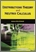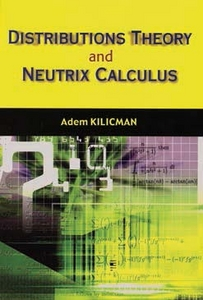Simple Search:

# Distribution theory and neutrix calculus

## Citation

Kilicman, Adem (2006) Distribution theory and neutrix calculus. Universiti Putra Malaysia Press, Serdang, Selangor. ISBN 9789833455395

## Abstract / Synopsis

Distributions also known as generalized functions which generalize classical functions and allow us to extend the concept of derivative to all continuous functions. The theory of distributions have applications in various fields especially in science and engineering where many non-continuous phenomena that naturally lead to differential equations whose solutions are distributions, such as the delta distribution therefore distributions can help us to develop an operational calculus in order to investigate linear ordinary differential equations as well as partial differential equations with constant coefficients through their fundamental solutions. Since theory of distributions is a linear theory, some regular operations which are valid for ordinary functions such as addition, multiplication by scalars are extended into distributions. Other operations can be defined only for certain restricted subclasses of distributions; these are called irregular operations. Certain divergent integrals can also be interpreted as distributions. Therefore our main aim in this monograph is to extend the above irregular operations to broader classes of distributions.

## Download FilePreview
PDF (Book cover)
Distribution theory and neutrix calculus.pdf

Download (23kB) | Preview

## Additional Metadata

Item Type: Book QA303 K48 Faculty of ScienceInstitute for Mathematical Research Universiti Putra Malaysia Press Distribution theory; Neutrix calculus Nadiana Adlia Rusli 24 Nov 2014 12:12 28 Jan 2015 15:37 http://psasir.upm.edu.my/id/eprint/32526 View Download Statistic

### Actions (login required)View Item